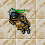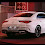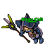## Sunday, April 3, 2022

### TibiaGoals Riddles E17R09

1.5+7 = 12

Skyelie Hasulhoff <3

2.Upper right vertical arrow of the number 9 goes on top of the - sign making it a + sign and the 9 changes to 5. 5+7=12

-Essiee

3.5+7=12
sir coor

4.5.6.7.Nick: Billy

8.Turn the 9 into 5 to make the - into +
5 + 7 = 12

Character Name: Dyaniixz

9.Turn the 9 into 5 to make the - into +
5 + 7 = 12

Character Name: Dyaniixz

10.Turn the 9 into 5 to make the - into +
5 + 7 = 12

Character Name: Dyaniixz

11.12.13.5+7=12 /Zatruty Szpon

14.9 - 7 = 12
9 7 ≠ 12

Nick: Iaaaaaaaaaaaa aaaaa Aaaaaaa

15.Alesita Sio

Se mueve la flecha del número 9 lado derecho superior formando asi el 5, esa misma flecha se pone sobre el signo - atravezandolo para formar el signo + y así obtenemos la suma 5+7 dando correctamente 12

5+7=12

16.9 - 7 = 12
5 + 7 = 12

Nick: Iaaaaaaaaaaaa aaaaa Aaaaaaa

17.5 + 7 = 12

18.5 + 7 = 12

Eve Esece

19.5+7=12
character name: pablosaurio

20.5+7=12
character name: pablosaurio

21.5+7=12
character name: pablosaurio

22.23.5+7=12

Xarkost Eilia

24.Meregirl

https://imgur.com/a/eBoLscO
5+7=12

25.26.5 + 7 = 12

RIGHO

27.5 + 7 =12
righo

28.5+7=12
RIGHO

29.5+7=12

Shizurie

30.5+7=12

Xafler

31.Presckot

The arrow above the right of the number "9", forming a sum, 5 + 7 = 12

32.33.9+7=12

Inkkz

34.5+7=12 ***

Inkkz

35.Psykeeh

5+7= 12

36.Grove Max. You need to move one arrow from the first digit (9) to the minus sign, changing it to +. We have 5 + 7 = 12

37.5+7=12; Lord Neganek

38.Tamarizz - Solidera

Move 1 arrow from the "9" to make the minus sign a plus sign, making the "9" a "5"
so the new equation would be:
5 + 7 = 12

39.Milny

Es 5+7=12
Se mueve una arrow de la parte superior del 9 para que forme el 5 y se completa el + con la arrow de la parte inferior.

40.Lyh

5 + 7 = 12;

41.5+7=12
Mian Stone'arrow

42.Answer: 5+7=12. We have to move 1 arrow from 9 to make +
Kenlux, Antica

43.I took a matchstick from 9 which turned it into a 5; and I put the matchstick vertically over the minus(-) to make it a plus(+).
so, 5+7=12

Paula Ines

44.move stick from 9 to make it 5 put it on the minus to make it plus

5 + 7 = 12

Toxic Hasulhoff

45.Grove Sorcerer, Move matchstick from nr. 9, change - to + witch this stick and we have 5 + 7 = 12

46.¯\_( ° -°)_/¯... How to explain which match you will take the best way... ¯\_( ° -°)_/¯

You take one match from the number 9 figure to make it into a sexy mambo number 5. Then you take the match that is left over and puts it on the negative figure "-" to make it into a plus sign "+". Then you get the wanted 5 + 7 = 12 equation.

8)

/Rand Silverfang

47.5+7=12. Name Puchi puchi

48.Kriiz Meow / Havera

https://imgur.com/oH7Drey

(5 + 7 = 12)

49.5 + 7 = 12 /Norelli

50.Heya! Move the top-right arrow of the 9 and use it to form a plus so the equation says 5+7=12.
Have a good start to your week! :D -Elyrea

51.5+7=12
righo

52.5+7=12
character Ewrr

53.5 + 7 = 12

Amy Meow

54.5+7
https://gyazo.com/bccd6f2e0f3da243b9abf33298e3d630

55.5+7=12

Nanka

56.5 + 7 = 12

Pameliin

57.5+7=12 Mikolaj

58.5+7=12 Mikolaj

59.5+7=12 Mikolaj

60.Panwierzba

5+7=12

61.R= 5+7 = 12

62.R= 5+7= 12
Samuray de carro :)

63.Congratulations to all participants!

You can control your results in our sheet: Riddles / Information

Remember, even if you got disqualified from the lottery, you can still participate in TibiaGoals riddles just for fun!

See you on Wednesday! Only one riddle left in current event!

64.